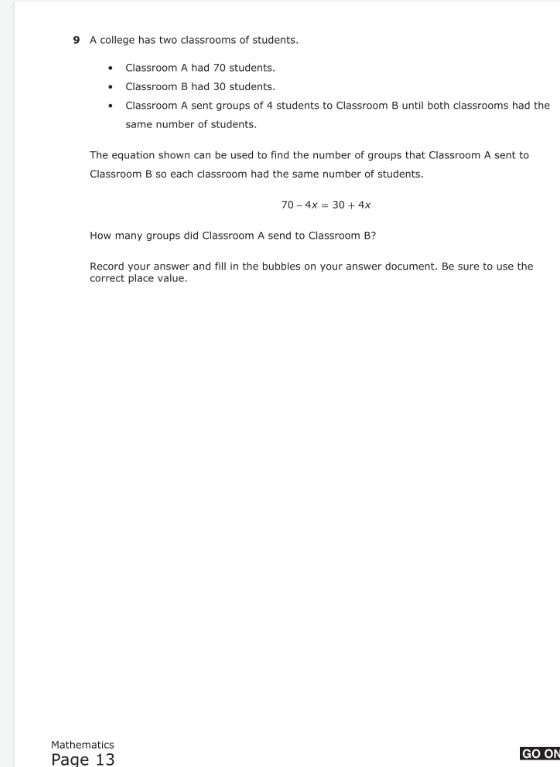### ¿Todavía tienes preguntas de matemáticas?

Pregunte a nuestros tutores expertos
Algebra
Pregunta$$9$$ A college has two classrooms of students.

- Classroom A had $$70$$ students.

- Classroom B had $$30$$ students. same number of students.

- Classroom A sent groups of $$4$$ students to Classroom B until both classrooms had the same number of students.

The equation shown can be used to find the number of groups that Classroom A sent to Classroom B so each classroom had the same number of students.

$$70 - 4 x = 30 + 4 x$$

How many groups did Classroom A send to Classroom B?